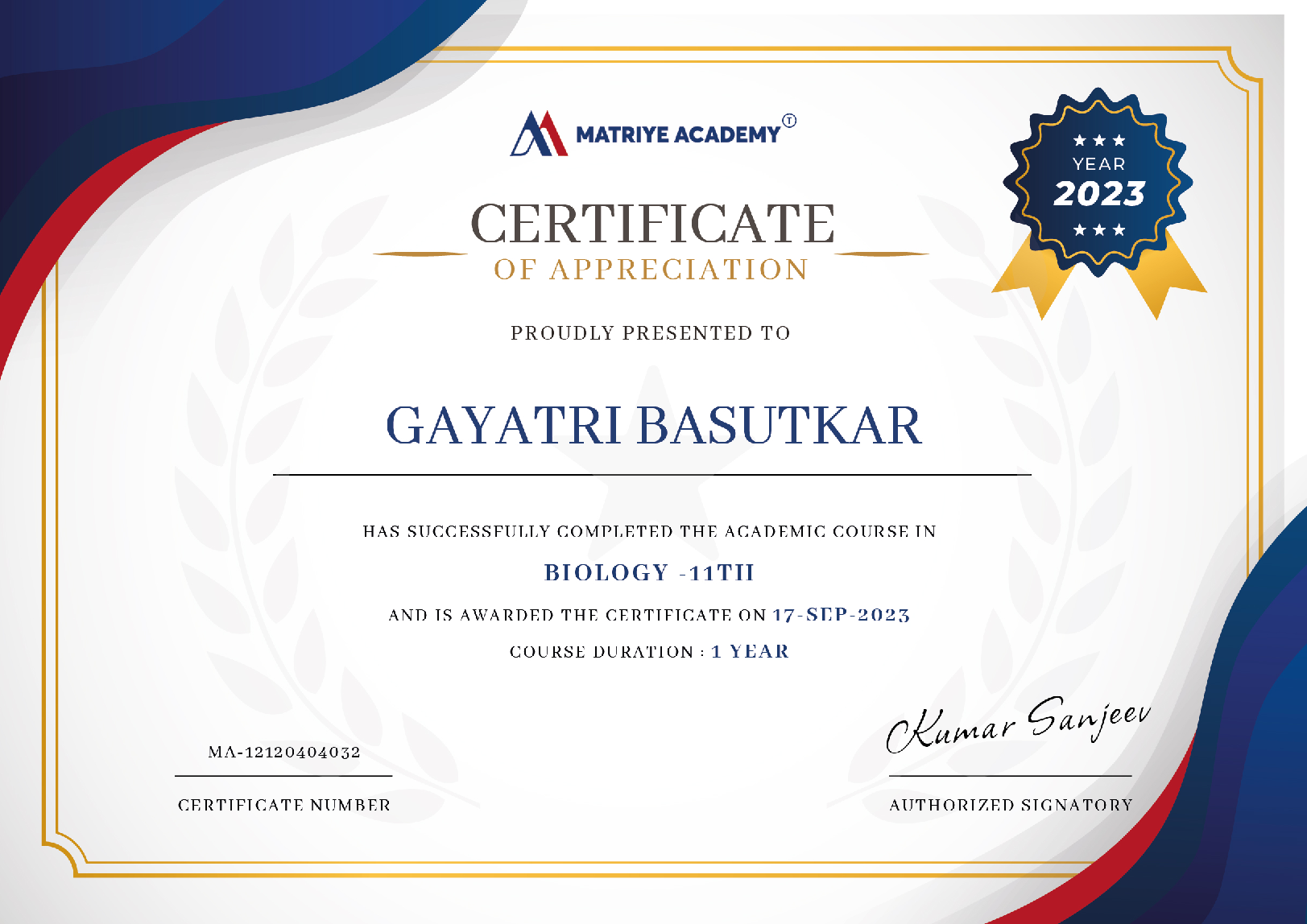# Math's

CBSE 6

The CBSE curriculum includes the subject of Mathematics so that CBSE Class 6 children may acquire analytical and problem-solving abilities##### Runali Deb Roy

Last Update : 07 Feb 2023

(4)

## Course Overview

Math is a high-scoring subject if you comprehend mathematical ideas. Identifying the curriculum at the start of the session will assist you in creating a study program.

Students will gain a better understanding of the topics thanks to the CBSE Class 6 Maths syllabus.

As you are aware, the CBSE Syllabus for Class 6 Mathematics is made up of 14 chapters, each with its own practice section. It is recommended that you go over the previous year's question papers and solve the equations. The practice will help you remember various concepts and improve your problem-solving speed.

### What You'll Learn

• Improves problem-solving speed and time management abilities.
• Understand the types of questions and the scoring system.
• Provides a sense of the real exam environment.
• Helps in determining the amount of preparedness.

14 Lessons 45m

### What is the CBSE syllabus for Class 6 Math?

The CBSE Syllabus For Class 6 Math introduces students to basic mathematical concepts. The syllabus begins with the chapter Knowing Our Numbers and concludes with the chapter Practical Geometry. The first section introduces students to various complex series of numbers, ranging from thousands to trillions.

### What topics are taught in 6th grade math?

6th Class Maths: The following are the various topics covered in the NCERT syllabus for 6th Class Maths: We are aware of our numbers. Numbers that are whole. I'm having fun with numbers. Geometrical fundamentals. Understanding elementary shapes. Integers.

### Class 6 Math's

Math is a subject that requires a lot of practice to truly understand the different types of problems that a student may be asked in an exam. It is critical to grasp the various sorts of issues presented in NCERT books and to practice solving them. Students can easily prepare for the annual exam by practicing the previous year's question papers and answering NCERT book questions. One can also overcome the constant worry that comes with studying for Math Exam.## 1

##### 1) Number System
5
5 ratings 4 reviews

1) Number System

(i) Knowing our Numbers

(ii) Playing with Numbers

(iii) Whole numbers

(iv) Negative Numbers and Integers

(v) Fractions

## 2

##### 2) Algebra
5
5 ratings 4 reviews

(i) Introduction to Algebra

### Course Instructor##### Shruti Koli
(5)
20 Tutorials 100 Students

It was the best experience I've ever had with it. It is an excellent resource for all students. Math is the best class, and you may quickly remove your worries.##### Ravikant Sharma
(5)
20 Tutorials 100 Students

The Matriye Academy website makes learning enjoyable and simple. Whenever I have a question, I just access the Matriye Academy website and look for the solution. It facilitates learning concepts through videos and courses.

### Start Learning Today

###### Financial aid available
• Taught by top companies and universities
• Affordable programs
• Apply your skills with hands-on projects
• Learn on your own schedule